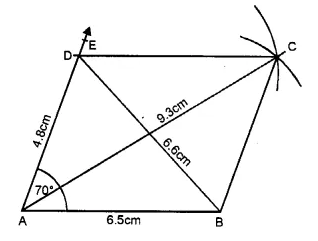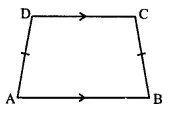## RS Aggarwal Class 6 Solutions Chapter 17 Quadrilaterals Ex 17A

These Solutions are part of RS Aggarwal Solutions Class 6. Here we have given RS Aggarwal Solutions Class 6 Chapter 17 Quadrilaterals Ex 17A

Other Exercises

Question 1.
Solution:
(i) Its diagonals are AC and BD
(ii) Two pairs of opposite sides are AB, CD and AD, BC
(iii) Two pairs of opposite angles are ∠A, ∠C and ∠B, ∠D
(iv) Two pairs of adjacent sides are AB, BC and CD and DA
(v) Two pairs of adjacent angles are ∠A, ∠B and ∠B, ∠C

Question 2.
Solution:
Steps of construction :
(i) Draw a line segment AB = 6.5 cm.(ii) At A, draw a ray AE making an angle of 70° with the help of the protractor and cut off AD = 4.8 cm.
(iii) With centre B and radius 4.8 cm and with centre D and radius 6.5 cm, draw two arcs intersecting each other at C.
(iv) Join BC and DC. Then ABCD is the required parallelogram.
(v) Join AC and BD which measures 9.3 cm and 6.6 cm respectively.

Question 3.
Solution:
Perimeter of the parallelogram = 56 cm
Ratio in sides = 4 : 3
Let first side = 4x
Then second side = 3x
Perimeter = 2 x sum of two sides
=> 56 = 2 x (4x + 3x)
=> 7x × 2 = 56
=> 14x = 56
=> x = $$\\ \frac { 56 }{ 14 }$$
= 4
First side = 4x = 4 × 4 = 16 cm and second side = 3x = 3 × 4 = 12 cm. Ans.

Question 4.
Solution:
(a) A parallelograms whose diagonals are equal and adjacent sides are unequal, is a rectangle.
(b) A parallelogram whose diagonal are equal and also side are equal, is a. square.
(c) A parallelogram whose diagonal are unequal but adjacent sides are equal is a rhombus.

Question 5.
Solution:
A quadrilateral whose one pair of opposite sides are equal but other pair non parallel, is called a trapezium
When the non-parallel sides of a trapezium are equal, then it is called an isosceles trapezium.
ABCD is an isosceles trapezium in which
Then ∠DAB = ∠CBA
On measuring, AD = BC = 3 cm
and ∠DAB = ∠CBA = 60°Question 6.
Solution:
(a) False , Diagonals of a parallelogram are not equal.
(b) False , Diagonals of a rectangle do not bisect each other at right angles.
(c) False , Diagonals of a rhombus are not equal.

Question 7.
Solution:
(a) Because if each side of a rectangle are equal it is called a square.
(b) Square is a special rhombus if its each angle is equal i.e., of 90°.
(c) If in a parallelogram, if each angle is of 90°, it is called a rectangle.
(d) A square is a parallelogram whose each side and each angle are equal.

Question 8.
Solution:
A regular quadrilateral is a quadrilateral if its each side and angles are equal square is a regular quadrilateral.

Hope given RS Aggarwal Solutions Class 6 Chapter 17 Quadrilaterals Ex 17A are helpful to complete your math homework.

If you have any doubts, please comment below. Learn Insta try to provide online math tutoring for you.